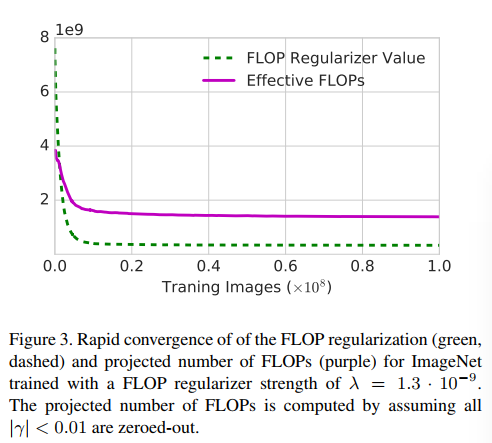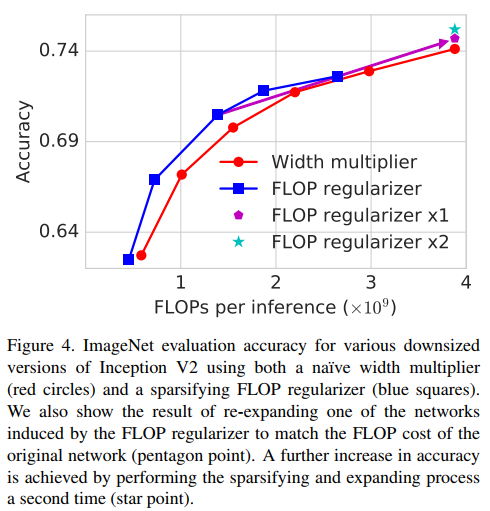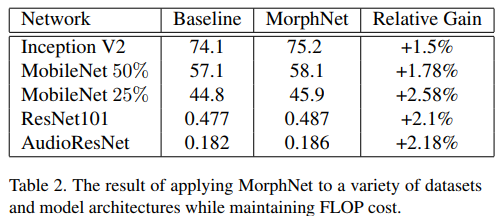### Introduction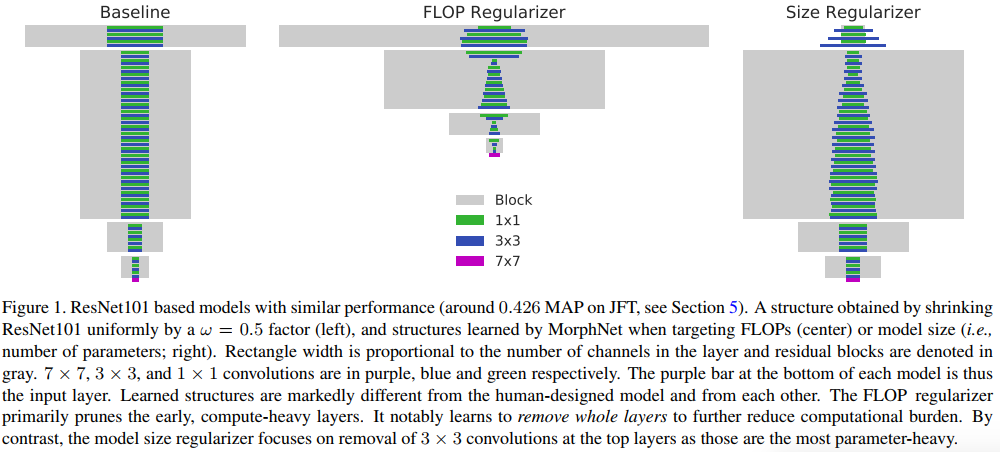MorphNet is an approach to automate the design of neural network structures. MorphNet iteratively shrinks and expands a network, shrinking via a resource weighted sparsifying regularizer on activations and expanding via a uniform multiplicative factor on all layers. The proposed method has 3 advantages :

• it is scalable to large models and large datasets;
• it can optimize a DNN structure targeting a specific resource, such as FLOPs per inference, while allowing the usage of untargeted resources such as model size (number of parameters), to grow;
• it can learn a structure that improves performance while reducing the targeted resource usage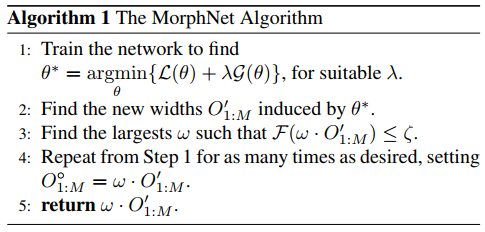### Method

Let $$O_L$$ be the output wight (number of output feature maps) or layer L and $$O_{1:M}$$ the total number of feature maps between the first and last layer (M is the index of the last layer). They formalized their problem as follows: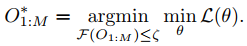where the goal is to find the most accurate model (with the lowest loss) but which respect a limited resource. For example here, the total number of flops must be below $$\zeta$$. They then reformulate the problem as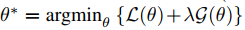where $$g(\theta)$$ is a sparsity regularizer which depends on $$F(O_{1:M} )$$. Since their method has no guarantee to satisfy $$F(O_{1:M})$$ < $$\zeta$$, they proposed Algo 1 where step 1 and 2 are compression steps and step 3 is an expansion step.

### The regularizer

The regularizer is given by the following formula: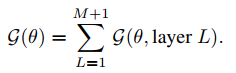where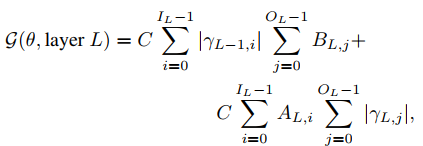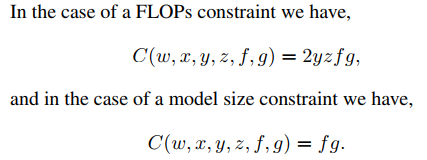and $$f,g$$ is the spatial dimension of the filter at layer L and $$x,z$$ the spatial dimension of the feature maps of layer L. Also, $$A_{L,i}$$ ($$B_{L,j}$$) is an indicator function which equals one if the i-th input (j-th output) of layer L is alive – not zeroed out.

### Results

And … the method works!